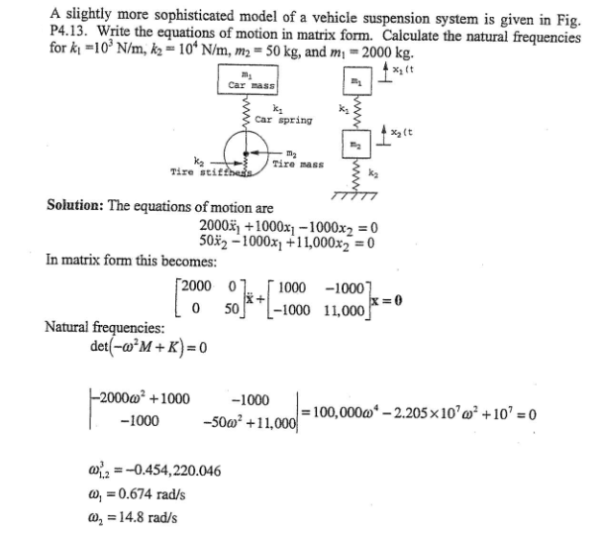A slightly more sophistticated model of a vehicle suspension system is given in the figure. Write the equations of motion in matrix form. Calculate the natural frequencies for k1=10^3 N/m, k2=10^4 N/m, m2=50 kg, and m1=2000 kg.A slightly more sophistticated model of a vehicle suspension system is given in the figure. Write the equations of motion in matrix form. Calculate the natural frequencies for k1=10^3 N/m, k2=10^4 N/m, m2=50 kg, and m1=2000 kg.

Vibrations Page 1 vibrations vibrations vibrations vibrations vibrations vibrations vibrations Vibrations Page 2